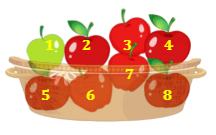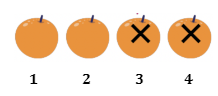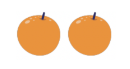Study P1 Mathematics Addition and Subtraction within 10 - Geniebook# Addition And Subtraction Within 10

In this article, we will focus on word problems based on addition and subtraction within 10. We will focus on:

2. Word problems involving subtraction
3. Word problems involving more than
4. Word problems involving less than

## 1. Word Problems Involving Addition

Example:

There are 3 girls and 2 boys in a park. How many children are there altogether?Solution:There are 3 girls and 2 boys. Therefore, the parts are 3 and 2.

The correct statement would be: $$3 + 2 = 5$$

There are 5 children altogether.

Question 1:

There are 5 bottles of strawberry milk and 4 bottles of chocolate milk. How many bottles of milk are there altogether?

1. 1
2. 4
3. 6
4. 9

(4) 9

Solution:

The given parts are 5 and 4. The total number of bottles will be $$5 + 4 = 9$$ bottles.

Question 2:

Joyce has 4 sweets. Linda has 6 sweets. How many sweets do they have altogether?

1. 6
2. 2
3. 10
4. 4

(3) 10

Solution:

The given parts are 4 and 6. The total number of sweets will be $$4 + 6 = 10$$

Question 3:

There are 4 books on a shelf. There are 2 books on a table. How many books are there altogether?

1. 6
2. 2
3. 8
4. 4

(1) 6

Solution:

The given parts are 4 and 2. The total number of sweets will be $$4 + 2 = 6$$

## Subtraction

Subtraction means taking away or finding the difference. Subtract means to minus ($$-$$). For example, $$10 - 2$$ can mean, “How many are left when you take 2 away from 10?” Alternatively, $$10 - 2$$ can also mean “How much more is 10 than 2?

## 2. Word Problems Involving Subtraction

Example:

There are 10 buttons in a box. There are 7 yellow buttons and the rest are orange buttons. How many orange buttons are there in the box?There are __________ orange buttons in the box.

3

Solution:

There are a total of 10 buttons in the box. If 7 buttons are yellow, the number of orange buttons in the box would be $$10 - 7 = 3$$.

In other words, we can say that:

$$\text{Whole} - \text{One Part} = \text{Other Part}$$

Question 1:

There are 8 apples in a basket. There is 1 green apple and the rest are red apples. How many red apples are there in the basket?1. 6
2. 7
3. 8
4. 9

(2) 7

Solution:

Let’s count the total number of apples in the basket.The whole will be 8. The given part will be 1 green apple. So, if we subtract this part from the whole, we will get the number of red apples:

$$8 - 1 = 7$$

Question 2:

There were 4 oranges on a table. Sam ate 2 oranges. How many oranges were left on the table?1. 5
2. 2
3. 6
4. 4

(2) 2

Solution:

There are 4 oranges in total. Sam ate 2 of them.We will subtract 2 oranges from 4 oranges to get the remaining number of oranges.The number of oranges left would then be:

$$4 - 2 = 2$$

Question 3:

Peter had 7 marbles. He gave away 2 marbles. How many marbles did Peter have left?1. 5
2. 2
3. 7
4. 4

(1) 5

Solution:

We will subtract 2 marbles from 7 marbles to get the remaining number of marbles Peter had left. Remember to subtract because Peter is giving away the marbles.

$$7 – 2 = 5$$

Question 4:

There were 10 balloons. 4 balloons burst. How many balloons were left?1. 3
2. 5
3. 6
4. 4

(3) 6

Solution:

Since 4 of the 10 balloons burst, we will subtract 4 from 10 to get the remaining number of balloons.

$$10 - 4 = 6$$

Question 5:

There were 6 butterflies in a garden. 3 of the butterflies flew away. How many butterflies were left?1. 1
2. 5
3. 3
4. 9

(3) 3

Solution:

3 butterflies flew away. This means that we need to subtract 3 from 6 to get the remaining number of butterflies.

$$6 - 3 = 3$$

Question 6:

Megan had 10 stickers. She lost 8 stickers. How many stickers did Megan have?1. 1
2. 2
3. 8
4. 10

(2) 2

Solution:

Since 8 out of 10 stickers were lost, we will subtract 8 from 10 in order to get the remaining stickers.

$$10 - 8 = 2$$

## 3. Word Problems Involving MORE THAN

Example:

May has 5 balloons. Ray has 2 more balloons than May. How many balloons does Ray have?7

Solution:

In order to find the number of balloons Ray has, we have to add 2 to the number of May’s balloons.

Therefore, $$5 + 2 = 7$$.

Question 1:

Nancy has 2 hamsters. Sara has 1 more hamster than Nancy. How many hamsters does Sara have?1. 1
2. 2
3. 3
4. 4

(3) 3

Solution:

Sara has 1 more hamster than Nancy. If Nancy has 2 hamsters, we have to add 1 to the number of hamsters that Nancy has to find Sara’s number of hamsters.

$$2 + 1 = 3$$

Question 2:

Joyce had 4 chocolates. She bought 6 more chocolates. How many chocolates does Joyce have in all?1. 6
2. 2
3. 10
4. 4

(3) 10

Solution:

In order to get the total number of chocolates that Joyce has, we will add the existing number of chocolates and the ones that she bought. The addition statement will be as follows:

$$4 + 6 = 10$$

Question 3:

Gary had 3 pencils. He bought 5 more pencils. How many pencils did Gary have altogether?

1. 8
2. 2
3. 3
4. 10

(1) 8

Solution:

In order to get the total number of pencils that Gary has, we will add the existing number of pencils and the ones that he bought. The addition statement will be as follows:

$$3 + 5 = 8$$

## 4. Word Problems Involving LESS THAN

Example:

John has 10 toy cars. Tommy has 2 less. How many toy cars does Tommy have?8

Solution:

The keyword in the question here is less, which means that we would need to subtract or minus. Since Tommy has 2 cars less than John, we will subtract 2 from 10 to get the answer.

$$10 - 2 = 8$$

Question 1:

Serene has 6 kites. Amanda has 1 less. How many kites does Amanda have?

1. 5
2. 6
3. 7
4. 8

(2) 5

Solution:

Since Amanda has 1 less kite than Serene, we will subtract 1 from 6 to get the number of kites Amanda has.

$$6 - 1 = 5$$

Question 2:

Angel has 9 Barbie dolls. Natalie has 2 fewer Barbie dolls than Angel. How many Barbie dolls does Natalie have?

1. 5
2. 6
3. 7
4. 8

(3) 7

Solution:

Since Natalie has 2 fewer than Angel, she will have $$9 - 2 = 7$$ Barbie dolls.

## Challenge Yourself !

Question 1:

There are 10 pens in a drawer. 2 of them are blue, 5 of them are red and the rest are green. How many green pens are there in the drawer?1. 3
2. 5
3. 7
4. 4

(1) 3

Solution:The total number of blue and red pens is $$2 + 5 = 7$$.

There are 10 pens in total. Therefore, we have to subtract 7 from 10 to get the number of green pens.

$$10 - 7 = 3$$

• Word problems involving subtraction
• Word problems involving more than
• Word problems involving less than

 Continue Learning Numbers To 10 Picture Graphs 1 Shapes 1 Ordinal Numbers Subtraction Within 20 Addition Within 10 Addition And Subtraction Within 10 Numbers To 20 Addition Within 20 Numbers To 100 Addition Within 100PrimaryPrimary 1EnglishMaths
Numbers To 10
Picture Graphs 1
Shapes 1
Ordinal Numbers
Subtraction Within 20
Numbers To 20
Numbers To 100Primary 2Primary 3Primary 4Primary 5Primary 6SecondarySecondary 1Secondary 2Secondary 3Secondary 4